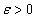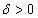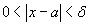# Epsilon Delta Limits Applet

This applet is designed to help users understand the epsilon/delta definition of a limit. The definition states that the limit of f(x) as x approaches a is L, and we writeif for every numberthere is a corresponding numbersuch thatwhenever. To test if the definition holds, the applet draws a horizontal band about f(x)=L in pink and yellow. This band contains all points (x,y) such that. A vertical band about x=a is shown in light green and yellow. This band contains all points (x,y) such that. Note that the yellow rectangle is the intersection of the delta and epsilon bands. If the limit is L, then for every point (x,f(x)) in the delta band (except possibly for x=a) must also lie in the epsilon band. An easy way of saying this is that if the definition holds, the graph of the function can't hit the green area.

The user can use mouse zooming to take a closer look. Click and drag to draw a box around the part of the graph that you want to magnify. Panning with right-click-and-drag is also enabled. For small values of epsilon and delta, you will want to zoom in on the point (a,L) and use the input boxes instead of the sliders to control the values of epsilon and delta. Several examples are configured with a and L set. The user may enter functions of this form in the "f(x)=" input box and choose values of a and L with the slider at the bottom of the applet.